# 3D-Gyro Simulation to control orientation of virtual object

Arduino Nano & 3-axis MEMS sensor (MPU-6050) takes real-time motion data and controls an orientation of virtual 3D cube in Processing IDE.

IntermediateFull instructions provided2 hours499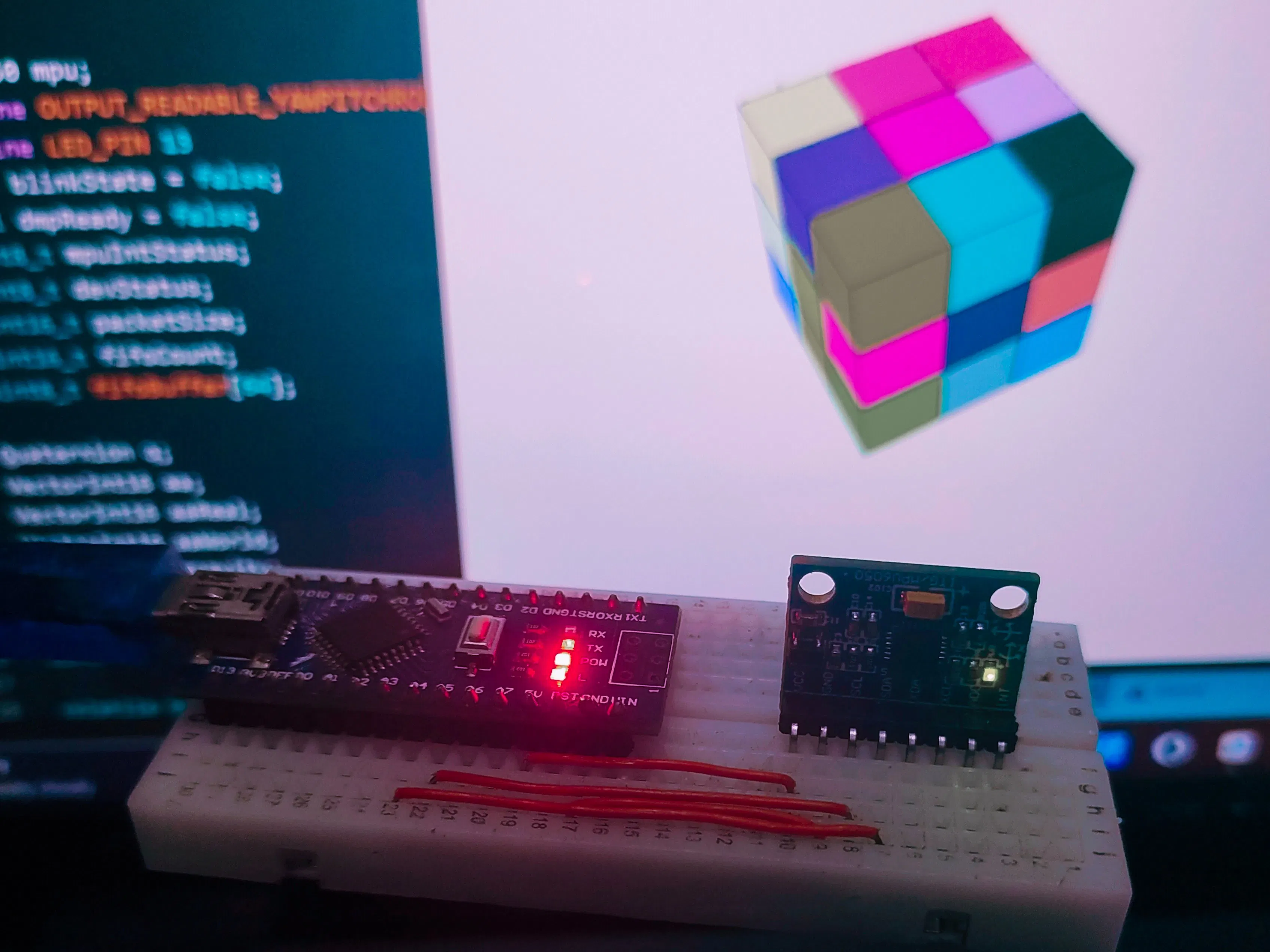## Things used in this project

### Hardware componentsArduino Nano R3
×1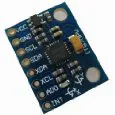Inertial Measurement Unit (IMU) (6 deg of freedom)
×1

### Software apps and online servicesArduino IDEThe Processing Foundation Processing

### Hand tools and fabrication machines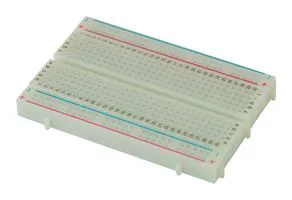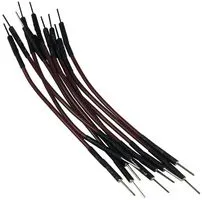10 Pc. Jumper Wire Kit, 5 cm Long

## Schematics

### Interfacing MPU-6050 with Arduino Nano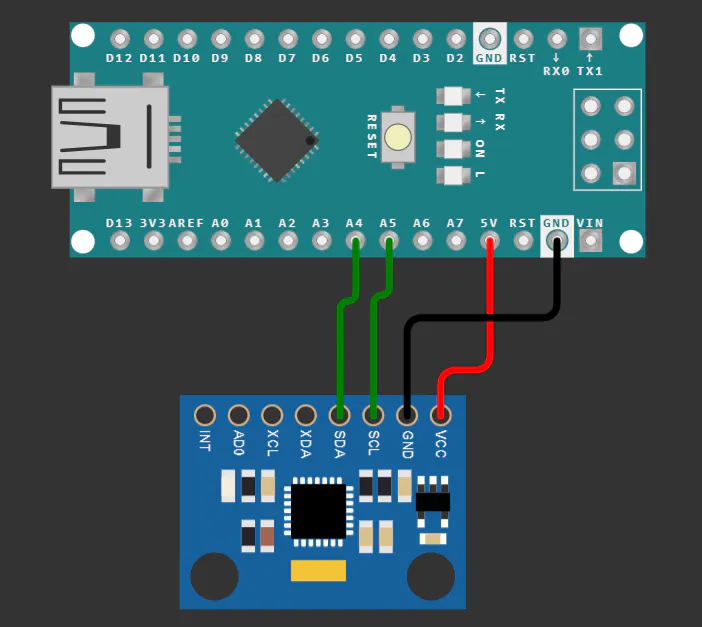## Code

### 3D-Gyro (Arduino IDE)

C/C++
```#include "I2Cdev.h"
#include "MPU6050_6Axis_MotionApps20.h"

#if I2CDEV_IMPLEMENTATION == I2CDEV_ARDUINO_WIRE
#include "Wire.h"
#endif

MPU6050 mpu;
#define LED_PIN 13
uint8_t mpuIntStatus;
uint8_t devStatus;
uint16_t packetSize;
uint16_t fifoCount;
uint8_t fifoBuffer;

Quaternion q;
VectorInt16 aa;
VectorInt16 aaReal;
VectorInt16 aaWorld;
VectorFloat gravity;
float euler;
float ypr;
uint8_t teapotPacket = { '\$', 0x02, 0,0, 0,0, 0,0, 0,0, 0x00, 0x00, '\r', '\n' };

volatile bool mpuInterrupt = false;
mpuInterrupt = true;
}

void setup() {

#if I2CDEV_IMPLEMENTATION == I2CDEV_ARDUINO_WIRE
Wire.begin();
TWBR = 24;
#elif I2CDEV_IMPLEMENTATION == I2CDEV_BUILTIN_FASTWIRE
Fastwire::setup(400, true);
#endif

Serial.begin(115200);
while (!Serial);
mpu.initialize();
devStatus = mpu.dmpInitialize();

mpu.setXGyroOffset(220);
mpu.setYGyroOffset(76);
mpu.setZGyroOffset(-85);
mpu.setZAccelOffset(1788);

if (devStatus == 0) {
mpu.setDMPEnabled(true);
mpuIntStatus = mpu.getIntStatus();
packetSize = mpu.dmpGetFIFOPacketSize();
} else {

Serial.print(devStatus);
Serial.println(F(")"));
}
pinMode(LED_PIN, OUTPUT);
}

void loop() {
while (!mpuInterrupt && fifoCount < packetSize) {
}

mpuInterrupt = false;
mpuIntStatus = mpu.getIntStatus();
fifoCount = mpu.getFIFOCount();

if ((mpuIntStatus & 0x10) || fifoCount == 1024) {
mpu.resetFIFO();

} else if (mpuIntStatus & 0x02) {
while (fifoCount < packetSize) fifoCount = mpu.getFIFOCount();
mpu.getFIFOBytes(fifoBuffer, packetSize);
fifoCount -= packetSize;

mpu.dmpGetQuaternion(&q, fifoBuffer);
Serial.print("quat\t");
Serial.print(q.w);
Serial.print("\t");
Serial.print(q.x);
Serial.print("\t");
Serial.print(q.y);
Serial.print("\t");
Serial.println(q.z);
#endif

mpu.dmpGetQuaternion(&q, fifoBuffer);
mpu.dmpGetEuler(euler, &q);
Serial.print("euler\t");
Serial.print(euler * 180/M_PI);
Serial.print("\t");
Serial.print(euler * 180/M_PI);
Serial.print("\t");
Serial.println(euler * 180/M_PI);
#endif

mpu.dmpGetQuaternion(&q, fifoBuffer);
mpu.dmpGetGravity(&gravity, &q);
mpu.dmpGetYawPitchRoll(ypr, &q, &gravity);
Serial.print(ypr * 18/M_PI);
Serial.print(" ");
Serial.print(ypr * 180/M_PI);
Serial.print(" ");
Serial.println(ypr * 180/M_PI);
#endif

mpu.dmpGetQuaternion(&q, fifoBuffer);
mpu.dmpGetAccel(&aa, fifoBuffer);
mpu.dmpGetGravity(&gravity, &q);
mpu.dmpGetLinearAccel(&aaReal, &aa, &gravity);
Serial.print("areal\t");
Serial.print(aaReal.x);
Serial.print("\t");
Serial.print(aaReal.y);
Serial.print("\t");
Serial.println(aaReal.z);
#endif

mpu.dmpGetQuaternion(&q, fifoBuffer);
mpu.dmpGetAccel(&aa, fifoBuffer);
mpu.dmpGetGravity(&gravity, &q);
mpu.dmpGetLinearAccel(&aaReal, &aa, &gravity);
mpu.dmpGetLinearAccelInWorld(&aaWorld, &aaReal, &q);
Serial.print("aworld\t");
Serial.print(aaWorld.x);
Serial.print("\t");
Serial.print(aaWorld.y);
Serial.print("\t");
Serial.println(aaWorld.z);
#endif

#ifdef OUTPUT_TEAPOT
teapotPacket = fifoBuffer;
teapotPacket = fifoBuffer;
teapotPacket = fifoBuffer;
teapotPacket = fifoBuffer;
teapotPacket = fifoBuffer;
teapotPacket = fifoBuffer;
teapotPacket = fifoBuffer;
teapotPacket = fifoBuffer;
Serial.write(teapotPacket, 14);
teapotPacket++;
#endif

}
}
```

### 3D-Gyro (Processing IDE)

Java
```import processing.serial.*;
import cc.arduino.*;

int W = 800;
int H = 800;

Serial port;
float Phi;
float Theta;
float Psi;

int cubeSize = 70;
int numCubes = 3;
int spacing = 2;

int[][][] cubeColors;

void setup() {
size(800, 800, P3D);
rectMode(CENTER);
smooth();
strokeCap(SQUARE);

background(255);

println(Serial.list());
port = new Serial(this, Serial.list(), 115200);
port.bufferUntil('\n');

cubeColors = new int[numCubes][numCubes][numCubes];

// Initialize the cube colors
for (int x = 0; x < numCubes; x++) {
for (int y = 0; y < numCubes; y++) {
for (int z = 0; z < numCubes; z++) {
cubeColors[x][y][z] = color(random(255), random(255), random(255));
}
}
}
}

void draw() {
background(255);
translate(W / 2, H / 2);

// Rotate the entire grid

// Draw the grid of cubes
for (int x = 0; x < numCubes; x++) {
for (int y = 0; y < numCubes; y++) {
for (int z = 0; z < numCubes; z++) {
pushMatrix();
translate((x - numCubes / 2) * (cubeSize + spacing),
(y - numCubes / 2) * (cubeSize + spacing),
(z - numCubes / 2) * (cubeSize + spacing));
fill(cubeColors[x][y][z]);
box(cubeSize);
popMatrix();
}
}
}
}

void serialEvent(Serial port)
{
if (input != null) {
input = trim(input);
String[] values = split(input, " ");
if (values.length == 3) {
float phi = float(values);
float theta = float(values);
float psi = float(values);
Phi = phi;
Theta = theta;
Psi = psi;
}
}
}
```

## Credits

### Anip Shah

9 projects • 2 followers
Practicing and learning about microcontroller boards and sensors.😊